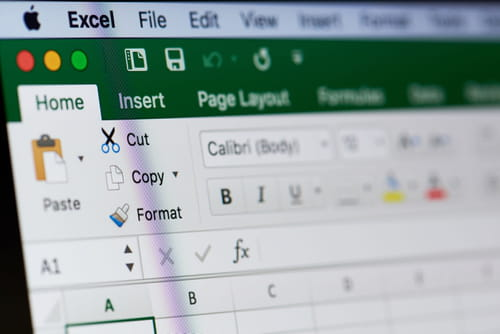15
Thanks

A few words of thanks would be greatly appreciated.

# How To Convert Numbers to Words in Excel

This article will teach you how to convert a numeric value in a Microsoft Excel worksheet cell into words using what is called the Spell Number function.## Convert Numbers to Words in Excel

The first method for converting a number to its written equivalent is to use direct entry. For example, if you were looking to write out 47.75 into forty seven dollars and seventy five cents, you can do this by entering the following formula into a cell:

`=SpellNumber(47.75)`

Another way to change numbers to text is to use Insert Function. To do this, select the cell that you want, and then, click Insert Function on the Formulas ribbon.

Under Or, select a category, followed by User Defined. In the Select a function list, select SpellNumber, followed by OK.

Next, enter the number or cell reference that you want, and then click OK.

Alternatively, you can also use the Concatenate function to apply this formula to specific cells (though this method is a bit more complicated). To use this function, enter the code below (be sure to replace B6 with the applicable cell number):

`=CONCATENATE(TEXT(UPPER(Spell Number(B6)),0),"ONLY")`

Image: © Dzmitry Kliapitski - Shutterstock.com

15
Thanks

A few words of thanks would be greatly appreciated.

CCM is a leading international tech website. Our content is written in collaboration with IT experts, under the direction of Jean-François Pillou, founder of CCM.net. CCM reaches more than 50 million unique visitors per month and is available in 11 languages.
Related
This document, titled « How To Convert Numbers to Words in Excel », is available under the Creative Commons license. Any copy, reuse, or modification of the content should be sufficiently credited to CCM (ccm.net).
2 vote - 3.0/5

trash
Posts
5
Registration date
Saturday March 21, 2015
Status
Member
Last seen
May 11, 2021
18
Thanks for tutorial.
I created to convert numbers to texts a function using VBA codes in Excel 2010 .Also , I added Dollars and Cents to last of words.

Function NumbertoText(ByVal MyNumber)
Dim Dollars, Cents, Temp As String
Dim DecimalPlace, Count
ReDim Place(9) As String
Place(2) = " Thousand "
Place(3) = " Million "
Place(4) = " Billion "
Place(5) = " Trillion "

' String representation of amount
MyNumber = Trim(Str(MyNumber))
' Position of decimal place 0 if none
DecimalPlace = InStr(MyNumber, ".")
'Convert cents and set MyNumber to dollar amount
If DecimalPlace > 0 Then
Cents = GetTens(Left(Mid(MyNumber, DecimalPlace + 1) & "00", 2))
MyNumber = Trim(Left(MyNumber, DecimalPlace - 1))
End If

Count = 1
Do While MyNumber <> ""
Temp = GetHundreds(Right(MyNumber, 3))
If Temp <> "" Then Dollars = Temp & Place(Count) & Dollars
If Len(MyNumber) > 3 Then
MyNumber = Left(MyNumber, Len(MyNumber) - 3)
Else
MyNumber = ""
End If
Count = Count + 1
Loop

Select Case Dollars
Case ""
Dollars = "No Dollars"
Case "One"
Dollars = "One Dollar"
Case Else
Dollars = Dollars & " Dollars"
End Select

Select Case Cents
Case ""
Cents = " and No Cents"
Case "One"
Cents = " and One Cent"
Case Else
Cents = " and " & Cents & " Cents"
End Select
NumbertoText = Dollars & Cents
End Function

' Converts a number from 100-999 into text
Private Function GetHundreds(ByVal MyNumber)
Dim Result As String
If Val(MyNumber) = 0 Then Exit Function
MyNumber = Right("000" & MyNumber, 3)
'Convert the hundreds place
If Mid(MyNumber, 1, 1) <> "0" Then
Result = GetDigit(Mid(MyNumber, 1, 1)) & " Hundred "
End If

'Convert the tens and ones place
If Mid(MyNumber, 2, 1) <> "0" Then
Result = Result & GetTens(Mid(MyNumber, 2))
Else
Result = Result & GetDigit(Mid(MyNumber, 3))
End If
GetHundreds = Result
End Function

' Converts a number from 10 to 99 into text. *
Private Function GetTens(TensText)
Dim Result As String
Result = "" 'null out the temporary function value
If Val(Left(TensText, 1)) = 1 Then ' If value between 10-19
Select Case Val(TensText)
Case 10: Result = "Ten"
Case 11: Result = "Eleven"
Case 12: Result = "Twelve"
Case 13: Result = "Thirteen"
Case 14: Result = "Fourteen"
Case 15: Result = "Fifteen"
Case 16: Result = "Sixteen"
Case 17: Result = "Seventeen"
Case 18: Result = "Eighteen"
Case 19: Result = "Nineteen"
Case Else
End Select
Else ' If value between 20-99
Select Case Val(Left(TensText, 1))
Case 2: Result = "Twenty "
Case 3: Result = "Thirty "
Case 4: Result = "Forty "
Case 5: Result = "Fifty "
Case 6: Result = "Sixty "
Case 7: Result = "Seventy "
Case 8: Result = "Eighty "
Case 9: Result = "Ninety "
Case Else
End Select
Result = Result & GetDigit _
(Right(TensText, 1)) 'Retrieve ones place
End If
GetTens = Result
End Function

' Converts a number from 1 to 9 into text.
Private Function GetDigit(Digit)
Select Case Val(Digit)
Case 1: GetDigit = "One"
Case 2: GetDigit = "Two"
Case 3: GetDigit = "Three"
Case 4: GetDigit = "Four"
Case 5: GetDigit = "Five"
Case 6: GetDigit = "Six"
Case 7: GetDigit = "Seven"
Case 8: GetDigit = "Eight"
Case 9: GetDigit = "Nine"
Case Else: GetDigit = ""
End Select
End Function

Details and sample file :https://www.technologicaltipstools.online/2016/11/excel-convert-numeric-value-into.html